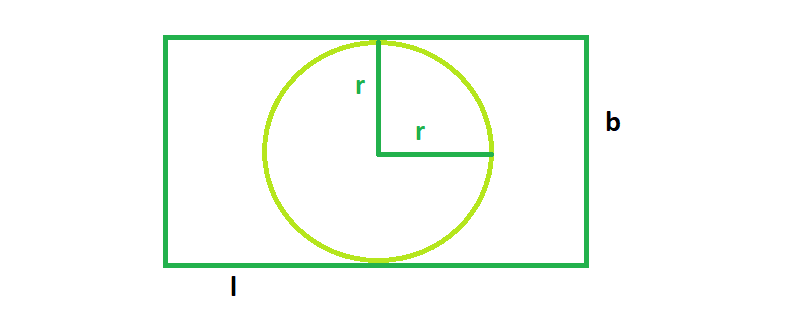Skip to content
Related Articles
The biggest possible circle that can be inscribed in a rectangle
• Last Updated : 15 Mar, 2021

Given a rectangle of length l & breadth b, we have to find the largest cricle that can be inscribed in the rectangle.
Examples:

```Input  : l = 4, b = 8
Output : 12.56

Input  : l = 16 b = 6
Output : 28.26```From the figure, we can see, the biggest circle that could be inscribed in the rectangle will have radius always equal to the half of the shorter side of the rectangle. So from the figure,

radius, r = b/2
Area, A = π * (r^2)

## C++

 `// C++ Program to find the biggest circle``// which can be inscribed  within the rectangle``#include ``using` `namespace` `std;` `// Function to find the area``// of the biggest circle``float` `circlearea(``float` `l, ``float` `b)``{` `    ``// the length and breadth cannot be negative``    ``if` `(l < 0 || b < 0)``        ``return` `-1;` `    ``// area of the circle``    ``if` `(l < b)``        ``return` `3.14 * ``pow``(l / 2, 2);``    ``else``        ``return` `3.14 * ``pow``(b / 2, 2);``}` `// Driver code``int` `main()``{``    ``float` `l = 4, b = 8;``    ``cout << circlearea(l, b) << endl;``    ``return` `0;``}`

## Java

 `// Java Program to find the``// biggest circle which can be``// inscribed within the rectangle` `class` `GFG``{` `// Function to find the area``// of the biggest circle``static` `float` `circlearea(``float` `l,``                        ``float` `b)``{` `// the length and breadth``// cannot be negative``if` `(l < ``0` `|| b < ``0``)``    ``return` `-``1``;` `// area of the circle``if` `(l < b)``    ``return` `(``float``)(``3.14` `* Math.pow(l / ``2``, ``2``));``else``    ``return` `(``float``)(``3.14` `* Math.pow(b / ``2``, ``2``));``}` `// Driver code``public` `static` `void` `main(String[] args)``{``    ``float` `l = ``4``, b = ``8``;``    ``System.out.println(circlearea(l, b));``}``}` `// This code is contributed``// by ChitraNayal`

## Python 3

 `# Python 3 Program to find the``# biggest circle which can be``# inscribed within the rectangle` `# Function to find the area``# of the biggest circle``def` `circlearea(l, b):` `    ``# the length and breadth``    ``# cannot be negative``    ``if` `(l < ``0` `or` `b < ``0``):``        ``return` `-``1` `    ``# area of the circle``    ``if` `(l < b):``        ``return` `3.14` `*` `pow``(l ``/``/` `2``, ``2``)``    ``else``:``        ``return` `3.14` `*` `pow``(b ``/``/` `2``, ``2``)` `# Driver code``if` `__name__ ``=``=` `"__main__"``:``    ``l ``=` `4``    ``b ``=` `8``    ``print``(circlearea(l, b))` `# This code is contributed``# by ChitraNayal`

## C#

 `// C# Program to find the``// biggest circle which can be``// inscribed within the rectangle``using` `System;` `class` `GFG``{` `// Function to find the area``// of the biggest circle``static` `float` `circlearea(``float` `l,``                        ``float` `b)``{` `// the length and breadth``// cannot be negative``if` `(l < 0 || b < 0)``    ``return` `-1;` `// area of the circle``if` `(l < b)``    ``return` `(``float``)(3.14 * Math.Pow(l / 2, 2));``else``    ``return` `(``float``)(3.14 * Math.Pow(b / 2, 2));``}` `// Driver code``public` `static` `void` `Main()``{``    ``float` `l = 4, b = 8;``    ``Console.Write(circlearea(l, b));``}``}` `// This code is contributed``// by ChitraNayal`

## PHP

 ``

## Javascript

 ``
Output:
`12.56`

Attention reader! Don’t stop learning now. Get hold of all the important DSA concepts with the DSA Self Paced Course at a student-friendly price and become industry ready.  To complete your preparation from learning a language to DS Algo and many more,  please refer Complete Interview Preparation Course.

In case you wish to attend live classes with industry experts, please refer Geeks Classes Live

My Personal Notes arrow_drop_up